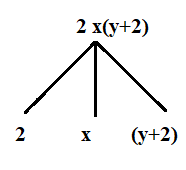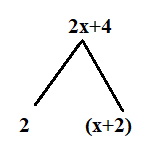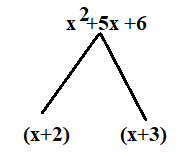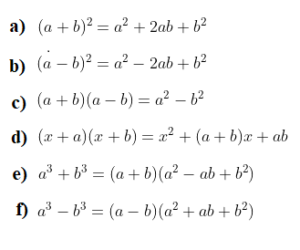December 8, 2023

# Factoring Polynomials Formula and Factoring Polynomials Worksheet with Answers

Factoring Polynomials Formula and Factoring Polynomials Worksheet with Answers

# What is Factorisation?

Representation of an algebraic expression  (  polynomials  )as the product of two or more expressions is called factorisation. Each such expression is called a factor of the given algebraic expression.  These factors may be numbers, algebraic variables or algebraic expressions.When  the factors  of the polynomial are multiplied together, you will get  the original polynomial.

## Methods of Factoring Polynomials

There are a certain number of methods by which we can factorise polynomials. Let us discuss these methods.

(i) Method of common factors

The first method for factoring polynomials will be factoring out the greatest common factor. It means look at all the terms and determine if there is a factor that is in common to all the terms. If there is, we will factor it out of the polynomial.  For example ,Suppose we have to factorise

. We shall write each term as a product of irreducible factors;
,  9 = 3 x 3 .  Hence

Now the  distributive law states that  a(b+c) = ab + bc . By using this law

Therefore, we can write

. Thus  factors of are  3 and
.

Note that we can always check our factoring by multiplying the terms back out to make sure we get the original polynomial.

Let’s take a look at some more examples.

Example 1:  Factorise    .

Solution :

Thus the common factors are and

i.e.  .

Therefore

.

Answer: Thus the factors of   are

and .

Example 2 : Factorise

.

Solution:

Thus the  common factors are 3 , 3 and i.e. 9y.

Therefore

.

and .

Example 3:  Factorise

.

Solution:

Thus the common factors are 3, a, b and c i.e. 3abc.

Therefore

Answer: Thus the factors of   are

and .

Example 4: Factorise

.

Solution :

Thus the common factors are and

i.e. .

Therefore

.

and
.

(ii)Factoring By Grouping

Let us try to understand grouping for factorizing with the help of the following examples.

Example 1:  Factorize the polynomial using the method of regrouping of factoring a polynomial.

Solution: For factoring polynomials we observe that we have no common factor among all the terms in the expression

. Let’s try regrouping them as and (6-9x).

are and
.

Example 2:  Factorise

Solution: There is no common factor among all the terms.  Notice that first two terms have a common factor

and the next two

terms have common factor  1.

So,

=

The factors of

are  and

Example 3 :  Factorise

Solution: There is no common factors in all the terms of

, So Grouping the terms, we have

=

Hence, the required factors  are

and .

Example 4: Factorise

Solution: Grouping the terms, we have

Hence, the required factors = and

Example 5: Factorise .

Solution:

=

=

=

Thus the required factors are

and .

(iii) Factorisation using  Algebraic Identities (Factoring Polynomials Formulas)

There are some nice special forms of some polynomials that can make factoring easier for us.  Some of them are given below.Example 1: Factorise  .

Sol.  The polynomial

. So,  it is of the form   where
and .

Now using the identity

we get,

.

Example 2: Factorise

.

Solution:   can be written as

. The expression is of the form   where
and ,

Now using the identity

we get,

=

Example 3: Factorise .

Solution:

.

The expression is of the form   where

and  ,

Now using the identity

we get,

=

Now, cannot be factorised further, but (

) is factorisable.

Again using the same identity , it follows that

.

Answer : Therefore  the factors of

are ,
and .

Example 4: Factorise

.

Solution:

=

[ Applying Identity with
]

=      [Applying Identity

with and
]

Answer  : Thus the factors of   are

and .

Example 5: Factorise

.

Solution: .

The expression is of the form

with and
.

Now applying the identity , we get

.

Answer : Therefore the factors of are

and .

Example 6: Factorise

.

Solution : . Now using the identity

,

we get  .

are and
.

### Factoring polynomial worksheet PDF

You might also be interested in:#### Bina singh

View all posts by Bina singh →
Last Minute Preparation Tips for SSC CGLTier II 2023 ssc chsl 2023 tier 1 cut off NIRF Rankings 2023 : Top 10 Engineering colleges in India CBSE Compartment Exam 2023 Application Form SSC CGL 2023 Notification: Important Dates